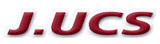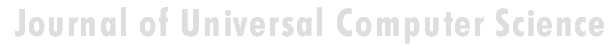Search
 Submission Procedure share: |available in:   PDF (224 kB) PS (328 kB)

get:
 Similar Docs BibTeX Write a comment
get:

DOI:   10.3217/jucs-016-18-2733

### Computable Separation in Topology, from T0 to T2

Klaus Weihrauch (University of Hagen, Germany)

Abstract: This article continues the study of computable elementary topology started in [Weihrauch and Grubba 2009]. For computable topological spaces we introduce a number of computable versions of the topological separation axioms T0, T1 and T2. The axioms form an implication chain with many equivalences. By counterexamples we show that most of the remaining implications are proper. In particular, it turns out that computable T1 is equivalent to computable T2 and that for spaces without isolated points the hierarchy collapses, that is, the weakest computable T0 axiom WCT0 is equivalent to the strongest computable T2 axiom SCT2. The SCT2-spaces are closed under Cartesian product, this is not true for most of the other classes of spaces. Finally we show that the computable version of a basic axiom for an effective topology in intuitionistic topology is equivalent to SCT2.

Keywords: axioms of separation, computable analysis, computable topology

Categories: F.0, F.m, G.0, G.m